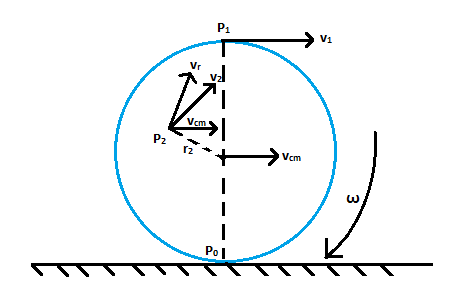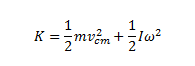# Rolling Motion | Formula & Diagram

In our day-to-day life, we observe various moving cars, bikes, rickshaws etc. All these circular wheels possess rolling motion. A body such as a sphere or a wheel or primarily a circular body that is rolling over a horizontal surface undergoes rolling motion and at every instant, there is a single point of contact. In the upcoming discussion, we will discuss rolling motion in detail. Let us assume that a disc-shaped body is rolling over a surface without slipping. In other words, at any particular instant of time, the part of the disc in contact with the surface is at rest with respect to the surface.

### Top Ten Questions of Rolling MotionThe rolling motion is a combination of translational motion and rotational motion. For a body, the motion of the centre of mass is the translational motion of the body. During the rolling motion of a body, the surfaces in contact get deformed a little temporarily. Due to this deformation, a finite area of both bodies comes in contact with each other. The overall effect of this phenomenon is that the component of the contact force parallel to the surface opposes motion resulting in friction.Let vcm is the velocity of the centre of mass of a disc-shaped body. Since for a rolling disc, the centre of mass would lie at the geometric centre C, the velocity of body or velocity of C is vcm which is parallel to the rolling surface. The rotational motion of the body occurs about its axis of symmetry, therefore, the velocity at any point P0, P1 or P2 of the body comprises two parts, translational velocity vcm and due to rotational motion, it has linear velocity vr, where vr = rω. ω is the angular velocity of the rolling disc. vr is perpendicular to radius vector at any point lying on the disc with respect to the geometric centre C. Consider the point P0 on the disc. vr is directed opposite to vcm and at this point vr = R ω , where R is the radius of the disc. Therefore, for the disc, the condition for rolling without slipping is given by vcm = R ω.

The kinetic energy of such a rolling body is given by the sum of kinetic energies of translational motion and rotation.Where,

m is the mass of the body

vcm is the rotational motion

I is the Moment of Inertia

ω is the angular velocity of the rolling body